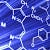# A very short introduction to Quick Sort Algorithm

Quick Sort Algorithm is a Divide and Conquer Algorithm. The basic concept of Quick Sort algorithm is we choose any element x, and we would be arranging the element x to is correct position. Correct position means that the elements left to x should be smaller than x and the elements right of x should be larger than x.

Let’s go through the pseudo code of quick sort function and the partition function.

## Pseudo Code of QuickSort() Function

`QuickSort(arr[], l, r){         if (l<r){                 int pi = partition (arr[], l, r)         QuickSort(arr[], l, pi-1)         QuickSort(arr[], pi+1, r)    }}`

You may also refer this GitHub Link…## Atindra Shekhar

I am currently in the sophomore year and pursuing Bachelors from Bennett University, India in Computer Science Engineering (CSE).